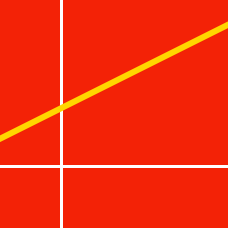Geometry

# Finding the Slope of a Line

Emma draws a line that has an equation $\frac{2y - 6}{36} = x$, what is the slope of the line?

What is the slope of the line passing through two points $P=(1, -30)$ and $Q=(5, -18)$?

Given a line $y = mx + 7$ that passes through the point $(6, 13 )$, what is the slope of the line, $m$?

If a line has slope $10$ and passes through the point $(3, 39)$, what value of $a$ in $(4, a)$ makes that point lie on the line?

Find the slope of a line that passes through the points $(2, -52)$ and $(8, 20)$.

×